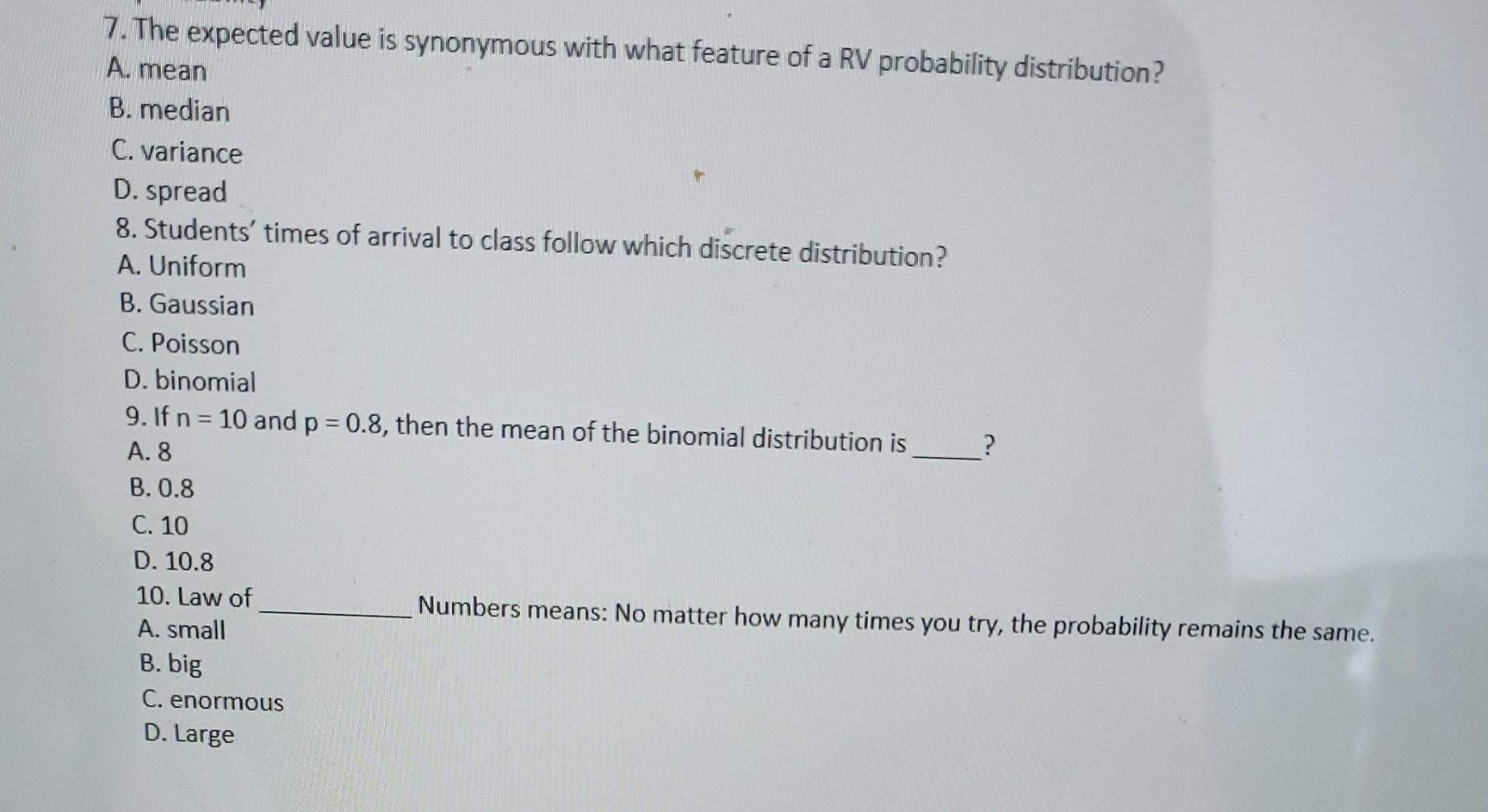# 7. The expected value is synonymous with what feature of a RV probability distribution?

7. The expected value is synonymous with what feature of a RV probability distribution? A. mean B. median C. variance D. spread 8. Students&#39; times of arrival to class follow which discrete distribution? A. Uniform B. Gaussian C. Poisson D. binomial 9. If n = 10 and p = 0.8, then the mean of the binomial distribution is ? A. 8 B. 0.8 C. 10 D. 10.8 10. Law of Numbers means: No matter how many times you try, the probability remains the same. A. small B. big C. enormous D. Large